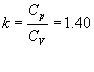# Theoretical and real cycles Cycles

## Cycles theoretical endothermic engines

For theoretical cycles, commonly used approaches aproximacióna by the real conditions are three:

• real cycle
• air cycle
• air-fuel cycle.

A theoretical these cycles are compared in practice the actual cycles, which are obtained experimentally by means of the indicators, for this reason, the actual cycle also indicated cycle is called.

Although the theoretical cycles do not correspond to actual cycles, provide a useful reference for the thermodynamic study of internal combustion engines, particularly to understand its use as they influence the operating conditions and to compare each other different types of engines.

## Theoretical Assumptions cycles

In the ideal cycles is assumed that the working fluid is constituted by air, and that it behaves like an ideal gas. Therefore, specific heat values â€‹â€‹are considered constant and equal to that of air at standard conditions of temperature 15 ° and 1 atmosphere pressure

Cp = 0.241 Ca / kg ° C;
Cv = 0.172 Ca / kg ° C;

Where is:Assume further that phases introduccióny heat removal are well-determined duration, dependent on the cycle type (Otto, Diesel, Sabathé) and that no other phases pé ; heat losses.

It is natural that, with these assumptions, the maximum values â€‹â€‹of temperature and pressure and, consequently, work and thermal efficiency calculated for the ideal cycle, are higher than for the other types of cycles.

The ideal cycle is, therefore, the maximum that can theoretically achieve the engine and allows easy mathematical study based on the perfect gas laws. He used we refer to throughout this agreement, the term "theoretical cycle".

## Air Cycle

In the air cycle, also working fluid is air, but it is assumed that the specific heats vary over the temperature range in which it operates.

Introduccióny conditions of heat removal equal the ideal cycle and there is no heat loss. Since the calculation of specific heats media is complicated tables that are used directly the values â€‹â€‹of the heat and work, in terms of internal energy and enthalpy for various points of the transformations Air isentropic. Considering the variations in specific heat are obtained for the high temperatures and pressures, lower values â€‹â€‹than those calculated for the ideal cycle, therefore, the work and thermal efficiency also lower but, nevertheless, are still higher than those for actual cycle.

The air-fuel cycle, including all those usually are calculated, the closest to the actual cycle. In the spark ignition engine, the fluid is made during the intake phase, by mixing and combustion gases above, in the compression ignition engine comprises air and the waste gases. After combustion, the fluid consists of the same product, namely a mixture of CO2, CO, H2O, N2. These gases have a mean specific heat still higher than that of air, but in addition, has a further increase in the specific heat due to the chemical decomposition disociacióno ; mica lighter molecules subjected to the action of high temperatures. The increase of specific heats, and their dissociation, being endothermic reaction absorbs some of the heat of combustion, producing a subsequent drop in temperature and Pressure paper , n maximum compared with those calculated for the air cycle.

For the calculation air-fuel cycle are used tables containing data obtained experimentally. Even for this cycle is supported not only that heat is added to and subtracted instantaneously, as in the ideal cycle but there is no loss of heat.

## real cycle combustion engines

The actual cycle is obtained experimentally, as mentioned above, through various display devices capable of recording the pressure diagram based on the volumes of a cylinder engine. The diagram shown reflects the actual conditions of the cycle and therefore takes into account variations in addition to the already set for the cycle of air and fuel-air cycles comparing ideal- heat losses, the combustion duration, losses caused by friction of the fluid, the time duration of valve opening, the ignition timing, as well as inyeccióny exhaust losses.

valoración: 2.7 - votos 12

Last review: February 1, 2016